# Mesh Parameterization¶

Important: Please read the installation page for details about how to install the toolboxes. $\newcommand{\dotp}{\langle #1, #2 \rangle}$ $\newcommand{\enscond}{\lbrace #1, #2 \rbrace}$ $\newcommand{\pd}{ \frac{ \partial #1}{\partial #2} }$ $\newcommand{\umin}{\underset{#1}{\min}\;}$ $\newcommand{\umax}{\underset{#1}{\max}\;}$ $\newcommand{\umin}{\underset{#1}{\min}\;}$ $\newcommand{\uargmin}{\underset{#1}{argmin}\;}$ $\newcommand{\norm}{\|#1\|}$ $\newcommand{\abs}{\left|#1\right|}$ $\newcommand{\choice}{ \left\{ \begin{array}{l} #1 \end{array} \right. }$ $\newcommand{\pa}{\left(#1\right)}$ $\newcommand{\diag}{{diag}\left( #1 \right)}$ $\newcommand{\qandq}{\quad\text{and}\quad}$ $\newcommand{\qwhereq}{\quad\text{where}\quad}$ $\newcommand{\qifq}{ \quad \text{if} \quad }$ $\newcommand{\qarrq}{ \quad \Longrightarrow \quad }$ $\newcommand{\ZZ}{\mathbb{Z}}$ $\newcommand{\CC}{\mathbb{C}}$ $\newcommand{\RR}{\mathbb{R}}$ $\newcommand{\EE}{\mathbb{E}}$ $\newcommand{\Zz}{\mathcal{Z}}$ $\newcommand{\Ww}{\mathcal{W}}$ $\newcommand{\Vv}{\mathcal{V}}$ $\newcommand{\Nn}{\mathcal{N}}$ $\newcommand{\NN}{\mathcal{N}}$ $\newcommand{\Hh}{\mathcal{H}}$ $\newcommand{\Bb}{\mathcal{B}}$ $\newcommand{\Ee}{\mathcal{E}}$ $\newcommand{\Cc}{\mathcal{C}}$ $\newcommand{\Gg}{\mathcal{G}}$ $\newcommand{\Ss}{\mathcal{S}}$ $\newcommand{\Pp}{\mathcal{P}}$ $\newcommand{\Ff}{\mathcal{F}}$ $\newcommand{\Xx}{\mathcal{X}}$ $\newcommand{\Mm}{\mathcal{M}}$ $\newcommand{\Ii}{\mathcal{I}}$ $\newcommand{\Dd}{\mathcal{D}}$ $\newcommand{\Ll}{\mathcal{L}}$ $\newcommand{\Tt}{\mathcal{T}}$ $\newcommand{\si}{\sigma}$ $\newcommand{\al}{\alpha}$ $\newcommand{\la}{\lambda}$ $\newcommand{\ga}{\gamma}$ $\newcommand{\Ga}{\Gamma}$ $\newcommand{\La}{\Lambda}$ $\newcommand{\si}{\sigma}$ $\newcommand{\Si}{\Sigma}$ $\newcommand{\be}{\beta}$ $\newcommand{\de}{\delta}$ $\newcommand{\De}{\Delta}$ $\newcommand{\phi}{\varphi}$ $\newcommand{\th}{\theta}$ $\newcommand{\om}{\omega}$ $\newcommand{\Om}{\Omega}$

This tour explores 2-D parameterization of 3D surfaces using linear methods.

A review paper for mesh parameterization can be found in:

M.S. Floater and K. Hormann, Surface Parameterization: a Tutorial and Survey in Advances in multiresolution for geometric modelling, p. 157-186, 2005.

K. Hormann, K. Polthier and A. Sheffer Mesh parameterization: theory and practice, Siggraph Asia Course Notes

In :
from __future__ import division

import numpy as np
import scipy as scp
import pylab as pyl
import matplotlib.pyplot as plt

from nt_toolbox.general import *
from nt_toolbox.signal import *
#from nt_solutions import meshdeform_1_parameterization as solutions

import warnings
warnings.filterwarnings('ignore')

%matplotlib inline


## Conformal Laplacian¶

The conformal Laplacian uses the cotan weights to obtain an accurate discretization of the Laplace Beltrami Laplacian.

They where first introduces as a linear finite element approximation of the Laplace-Beltrami operator in:

U. Pinkall and K. Polthier, Computing discrete minimal surfaces and their conjugates Experimental Mathematics, 2(1):15-36, 1993.

First load a mesh. The faces are stored in a matrix $F = (f_j)_{j=1}^m \in \RR^{3 \times m}$ of $m$ faces $f_j \in \{1,\ldots,n\}^3$. The position of the vertices are stored in a matrix $X = (x_i)_{i=1}^n \in \RR^{3 \times n}$ of $n$ triplets of points $x_k \in \RR^3$

In :
from nt_toolbox.read_mesh import *

n = np.shape(X)


In order to perform mesh parameterization, it is important that this mesh has the topology of a disk, i.e. it should have a single B.

First we compute the boundary $B = (i_1,\ldots,i_p)$ of the mesh. By definition, for the edges $(i_k,i_{k+1})$, there is a single adjacent face $(i_k,i_{k+1},\ell)$.

In :
from nt_toolbox.compute_boundary import *
B = compute_boundary(F)


Length of the boundary.

In :
p = len(B)


Display the boundary.

In :
from nt_toolbox.plot_mesh import *

plt.figure(figsize=(10,10))
#plot mesh
plot_mesh(X, F, el=80, az=-100, lwdt=.6, dist=6)

#plot boundary
for i in range(len(B)):
plt.plot(X[0,B], X[1,B], X[2,B], color="red", lw=3)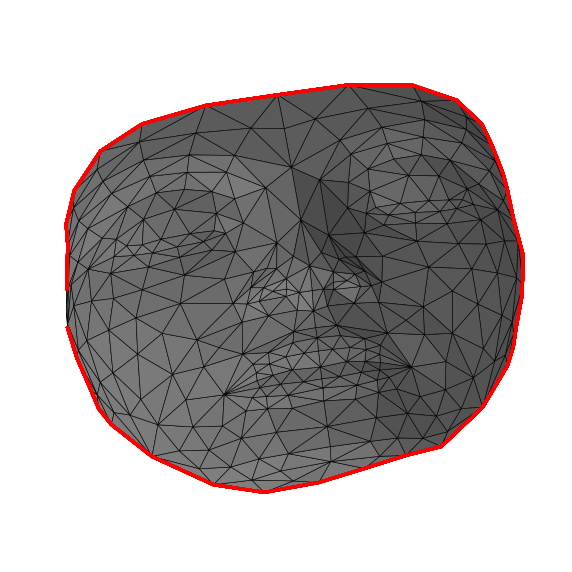The conformal Laplacian weight matrix $W \in \RR^{n \times n}$ is defined as $$W_{i,j} = \choice{ \text{cotan}(\al_{i,j}) + \text{cotan}(\be_{i,j}) \qifq i \sim j \\ \quad 0 \quad \text{otherwise}. }$$ Here, $i \times j$ means that there exists two faces $(i,j,k)$ and $(i,j,\ell)$ in the mesh (note that for B faces, one has $k=\ell$).

The angles are the angles centered as $k$ and $\ell$, i.e. $$\al_{i,j} = \widehat{x_i x_k x_j } \qandq \be_{i,j} = \widehat{x_i x_\ell x_j }.$$

Compute the conformal 'cotan' weights. Note that each angle $\alpha$ in the mesh contributes with $1/\text{tan}(\alpha)$ to the weight of the opposite edge. We compute $\alpha$ as $$\alpha = \text{acos}\pa{ \frac{\dotp{u}{v}}{\norm{u}\norm{v}} }$$ where $u \in \RR^3, v \in \RR^3$ are the edges of the adjacent vertices that defines $\al$.

In :
W = sparse.coo_matrix(np.zeros([n,n]))

for i in range(3):
i2 = (i+1)%3
i3 = (i+2)%3
u = X[:,F[i2,:]] - X[:,F[i,:]]
v = X[:,F[i3,:]] - X[:,F[i,:]]
# normalize the vectors
u = u/np.tile(np.sqrt(np.sum(u**2,0)), (3,1))
v = v/np.tile(np.sqrt(np.sum(v**2,0)), (3,1))
# compute angles
alpha = 1/np.tan(np.arccos(np.sum(u*v, 0)))
alpha = np.maximum(alpha, 1e-2*np.ones(len(alpha))) #avoid degeneracy
W = W + sparse.coo_matrix((alpha,(F[i2,:],F[i3,:])),(n,n))
W = W + sparse.coo_matrix((alpha,(F[i3,:],F[i2,:])),(n,n))


Compute the symmetric Laplacian matrix $L = D-W$ where $D = \mathrm{Diag}_i\pa{\sum_j W_{i,j}}$

In :
d = W.sum(0)
D = sparse.diags(np.ravel(d),0)
L = D - W


## Fixed Boundary Harmonic Parameterization¶

The problem of mesh parameterization corresponds to finding 2-D locations $(y_i = (y_i^1,y_i^2) \in \RR^2$ for each original vertex, where $Y = (y_i)_{i=1}^n \in \RR^{2 \times n}$ denotes the flattened positions.

The goal is for this parameterization to be valid, i.e. the 2-D mesh obtained by replacing $X$ by $Y$ but keeping the same face connectivity $F$ should not contained flipped faces (all face should have the same orientation in the plane).

We consider here a linear methods, that finds the parameterization, that impose that the coordinates are harmonic inside the domain, and have fixed position on the boundary (Dirichlet conditions) $$\forall s=1,2, \quad \forall i \notin B, \quad (L y^s)_i = 0, \qandq \forall j \in B, \quad y^s_j = z_j^s.$$

In order for this method to define a valid parameterization, it is necessary that the fixed position $z_j = (z^1_j,z^2_j) \in \RR^2$ are consecutive points along a convex polygon.

Compute the fixed positions $Z=(z_j)_j$ for the vertices on $B$. Here we use a circle.

In :
p = len(B)
t = np.linspace(0,2*np.pi,p+1)
t = np.delete(t,p)
Z = np.vstack((np.cos(t),np.sin(t)))


Computing the parameterization requires to solve two independent linear system $$\forall s=1,2, \quad L_1 y^s = r^s$$ where $L_1$ is a modified Laplacian, the is obtained from $L$ by $$\choice{ \forall i \notin B, \quad (L_0)_{i,j} = L_{i,j} \\ \forall i \in B, \quad (L_0)_{i,i}=1, \\ \forall i \in B, \forall j \neq i, \quad (L_0)_{i,i}=0, }$$ i.e. replacing each row indexed by $B$ by a 1 on the diagonal.

In :
L1 = np.copy(L.toarray())
L1[B,:] = 0
for i in range(len(B)):
L1[B[i], B[i]] = 1
L1 = sparse.coo_matrix(L1)


Set up the right hand size $R$ with the fixed position.

In :
R = np.zeros([2,n])
R[:,B] = Z


Solve the two linear systems.

In :
from scipy.sparse import linalg

Y = np.zeros([2,n])
Y[0,:] = linalg.spsolve(L1, R[0,:])
Y[1,:] = linalg.spsolve(L1, R[1,:])


Display the parameterization.

In :
plt.figure(figsize=(10,10))
plot_mesh(np.vstack((Y,np.zeros(n))),F, lwdt=1, c="lightgrey")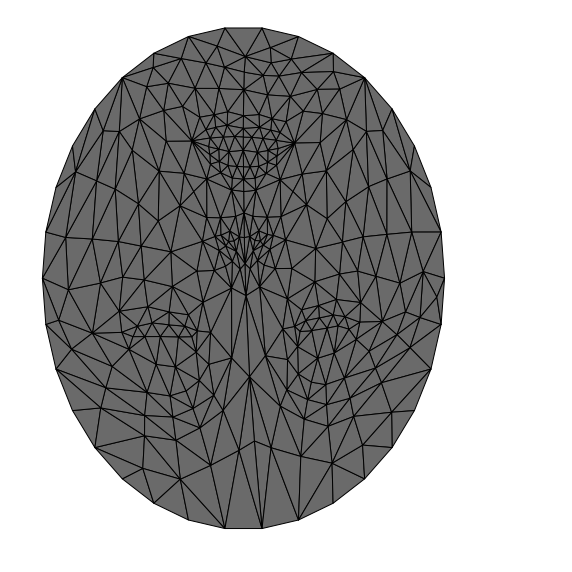## Mesh Parameterization on a Square¶

One can perform a fixed B parameterization on a square. This is useful to compute a geometry image (a color image storring the position of the vertices).

Exercise 1

Compute the fixed positions $Z$ of the points indexed by $B$ that are along a square. Warning: $p$ is not divisible by 4.

In :
run -i nt_solutions/meshdeform_1_parameterization/exo1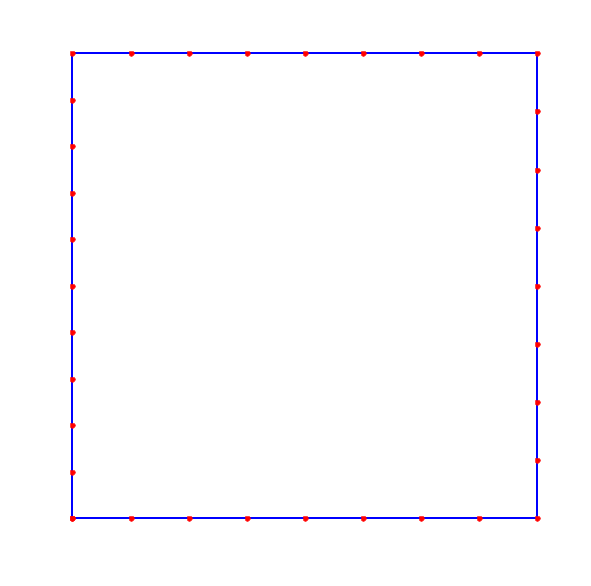In :
## Insert your code here.


Exercise 2

Compute the parameterization $Y$ on a square.

In :
run -i nt_solutions/meshdeform_1_parameterization/exo2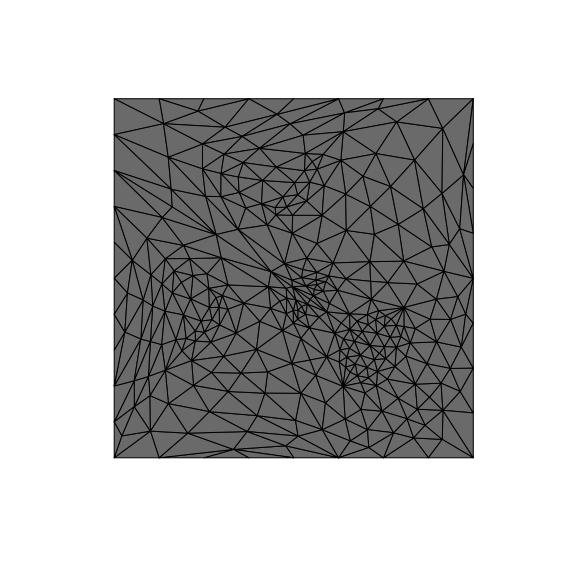In :
## Insert your code here.


Exercise 3

Shift the $B$ positions so that the eyes of the model are approximately horizontal.

In :
run -i nt_solutions/meshdeform_1_parameterization/exo3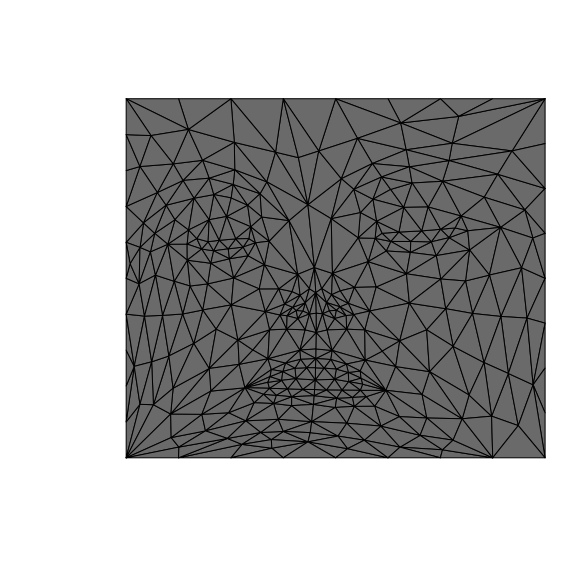In :
## Insert your code here.


## Re-align the Texture¶

To map correctly a real image on the surface, the texture needs to be aligned. We use here a simple affine mapping to map the eye and mouth of the image on the corresponding location on the surface.

Load a texture image $T$.

In :
from numpy import random

C = random.rand(1000,3)
C = C/np.repeat(np.sum(C,1)[:,np.newaxis],3,1)
C[np.lexsort((C[:,2],C[:,1],C[:,0]))]

lambd=np.transpose(C)

In :
n1 = 256
T = T[::-1,:]


Display the texture on the mesh, using the parametrization of the mesh as texture coordinates.

In :
from mpl_toolkits.mplot3d import Axes3D
from mpl_toolkits.mplot3d import art3d
from scipy import interpolate

fig = plt.figure(figsize = (10,10))

x = np.linspace(0, 1, np.shape(T))
f = interpolate.RectBivariateSpline(x, x, np.transpose(T))

for i in range(np.shape(F)):

px = Y[0,F[:,i]]
py = Y[1,F[:,i]]

points_x = np.dot(np.transpose(lambd),px[:,np.newaxis])
points_y = np.dot(np.transpose(lambd),py[:,np.newaxis])
points = np.hstack((points_x,points_y))

col = rescale(f.ev(points_x, points_y))
col = np.repeat(col,3,1)

Px = X[0,F[:,i]]
Py = X[1,F[:,i]]
Pz = X[2,F[:,i]]

Points_x = np.dot(np.transpose(lambd),Px[:,np.newaxis])
Points_y = np.dot(np.transpose(lambd),Py[:,np.newaxis])
Points_z = np.dot(np.transpose(lambd),Pz[:,np.newaxis])

ax.scatter(Points_x,Points_y,Points_z,c=col,s=5,lw=0,alpha=1)
ax.view_init(elev=80, azim=-100)
ax.axis("off")
ax.dist=5